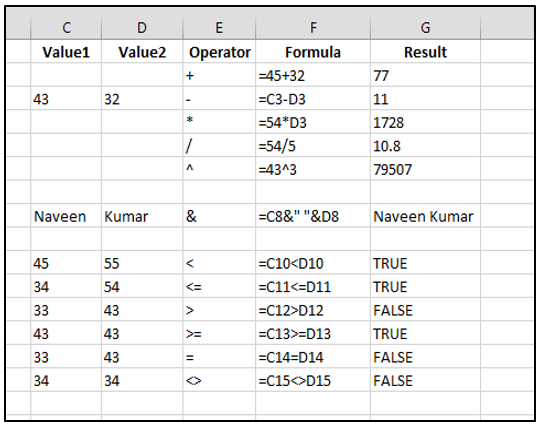Formulas and Functions in Excel 2013Formula and Function in Excel 2013

Formula : - A formula is an equation used to perform a calculation on given numbers, cell references or operators. In a formula, may contains Numbers, cell-references, Mathematical (+,  – ,  * ,  / ,  ^), Conditional operator (<,  <=, >, >=,  =,  <>). It is look like a mathematical expression. Ex- =A1+B1+C1 – D1*5/2

Properties of Formula-

A Formula must be started with (=) equal to sign.

A Formula contains a number, mathematical expression, operator, cell reference, parenthesis-(), etc.

A formula that cannot contains self-cell-reference.

When you press enter key after entering formula in a cell, only displayed result of formula in the cell, formula displayed in the formula bar.

Operator: - Operator is a symbol, to operate the given operand. It operate the operand according to nature of operator. Three types of operator are given below, which are mostly used in excel 2013.

 Arithmetic Operator + Addition – Subtraction * Multiplication / Division ^ Exponent

 Text Operator & Join of two or more text.Conditional Operator > Greater than < Less than >= Greater than or equal to <= Less than or equal to = Equal to <> Not equal to

Functions – A predefined formula is called a function. A function is used for calculate a simple or complex calculation in easy way. A function requires necessary arguments to calculate/execute.

Types of functions –

1-    Arithmetic Function

Ex-  Sum(), abs(), round(), trunc(), log(), pi(), etc.

2-    Statistical Function

Ex- Average(), count(), max(), min(), etc.

3-    Text function

Ex- Concatenate(), Left(), Right(), Upper(), Lower(), Proper(), Find(), etc.

4-    Date/Time Function

Ex- Now(), today(), day(), month(), year(), datevalue(), days(), etc.

5-    Logical Function.

Ex- if(), sumif(), countif(), and(), or(), not(), etc.

6-    Financial Function.

Ex- pmt(), pv(), fv(), intrate(), etc.